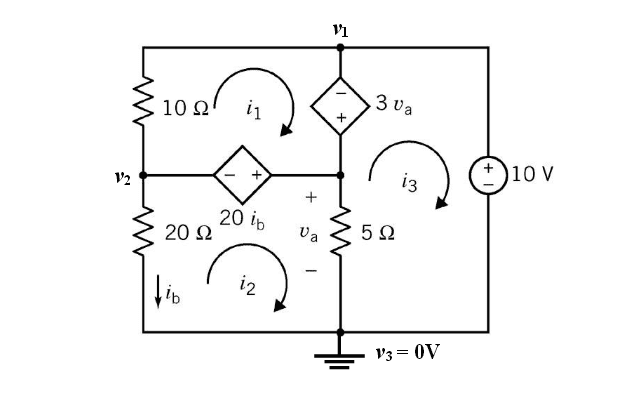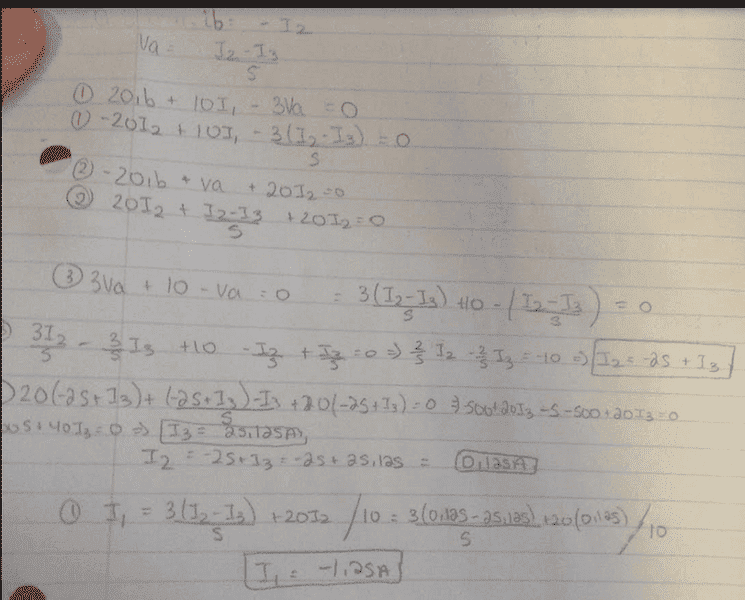# Mesh analysis with dependent sources

• Cocoleia

## Homework Statement

I need to use mesh analysis to find the three currents:## The Attempt at a SolutionThe answers are I1= -1.25A, I2=0.125A and I3 = 1.125A
I don't know where I messed up for I3

## Homework Statement

I need to use mesh analysis to find the three currents:
View attachment 110493

## The Attempt at a Solution

View attachment 110491
The answers are I1= -1.25A, I2=0.125A and I3 = 1.125A
I don't know where I messed up for I3
How di you get Va = (I2-I3)/5 ?

How di you get Va = (I2-I3)/5 ?
It should be multiplied

from the 5Ω resistance
My point is that a voltage is a resistance times a current, it cannot be a current divided by a resistance

My point is that a voltage is a resistance times a current, it cannot be a current divided by a resistance
Thank you I didn't realize my error